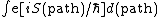# Feynman integral

## Feynman integral

A technique, also called the sum over histories, which is basic to understanding and analyzing the dynamics of quantum systems. It is named after fundamental work of Richard Feynman. The crucial formula gives the quantum probability density for transition from a point q0 to a point q1 in time t as the expression below, where S(path)is the classical mechanical action of a trial path, and &planck; is the rationalized Planck's constant. The integral is a formal one over the infinite-dimensional space of all paths which go from q0 to q1 in time t. Feynman defines it by a limiting procedure using approximation by piecewise linear paths.

Feynman integral ideas are especially important in quantum field theory, where they not only are a useful device in analyzing perturbation series but are also one of the few nonperturbative tools available. See Quantum field theory

An especially attractive element of the Feynman integral formulation of quantum dynamics is the classical limit, &planck; → 0. Formal application of the method of stationary phase to the above expression says that the significant paths for small &planck; will be the paths of stationary action. One thereby recovers classical mechanics in the hamiltonian stationary action formulation. See Least-action principle

McGraw-Hill Concise Encyclopedia of Physics. © 2002 by The McGraw-Hill Companies, Inc.

## Feynman integral

[′fīn·mən ′int·ə·grəl]
(quantum mechanics)
A term in a perturbation expansion of a scattering matrix element; it is an integral over the Minkowski space of various particles (or over the corresponding momentum space) of the product of propagators of these particles and quantities representing interactions between the particles.
McGraw-Hill Dictionary of Scientific & Technical Terms, 6E, Copyright © 2003 by The McGraw-Hill Companies, Inc.
Mentioned in ?
References in periodicals archive ?
The corresponding expression of the Feynman integral is
Johnson, "The equivalence of two approaches to the Feynman integral," Journal of Mathematical Physics, vol.
In perturbative QFT, one has to consider Feynman graphs, and to associate to each such a graph a Feynman integral (further related to quantities actually measured in physical experiments).
Thus the fractional QM (FQM) based on the Levy path integral generalizes the standard QM based on the Feynman integral for example.
Among those topics are processes in Euclidean spaces, heat kernels for stable-like processes, and complex Markov chains and Feynman integral.
This phenomenon is very much like the case of Feynman integral, which had been regarded as a finitely additive measure with unbounded total variation before the theory of white noise was introduced by T.
Then similarly we have the Feynman rules such that the one-loop electron self-energy is given by the following Feynman integral:
Similarly we have the Feynman rules that the one-loop vertex correction is given by the following Feynman integral:
Among the topics are integrable lattice models from four-dimensional field theories, the physics and the mixed Hodge structure of Feynman integrals, a quick guide to defect orbifolds, Calabi-Yau fourfolds in products of projective space, strict deformation quantization of the G-connections via lie groupoid, and instanton-soliton loops in five-dimensional super-Yang-Mills.
They consider such topics as the uniqueness of bounded solutions to aggregation equations by optimal transport methods, effective equidistribution and spectral gap, positive definite functions in distance geometry, topological field theories in two dimensions, Brouwer's dissertation and the revolution of 1907, new developments in combinatorial number theory and applications, geometry and non-archimedian integrals, Feynman integrals and motives, computational complexity and numerical stability of linear problems, and high-dimensional distributions with convexity properties.
Srivastava, The [bar.H]-function associated with a certain class of Feynman integrals, J.

Site: Follow: Share:
Open / Close